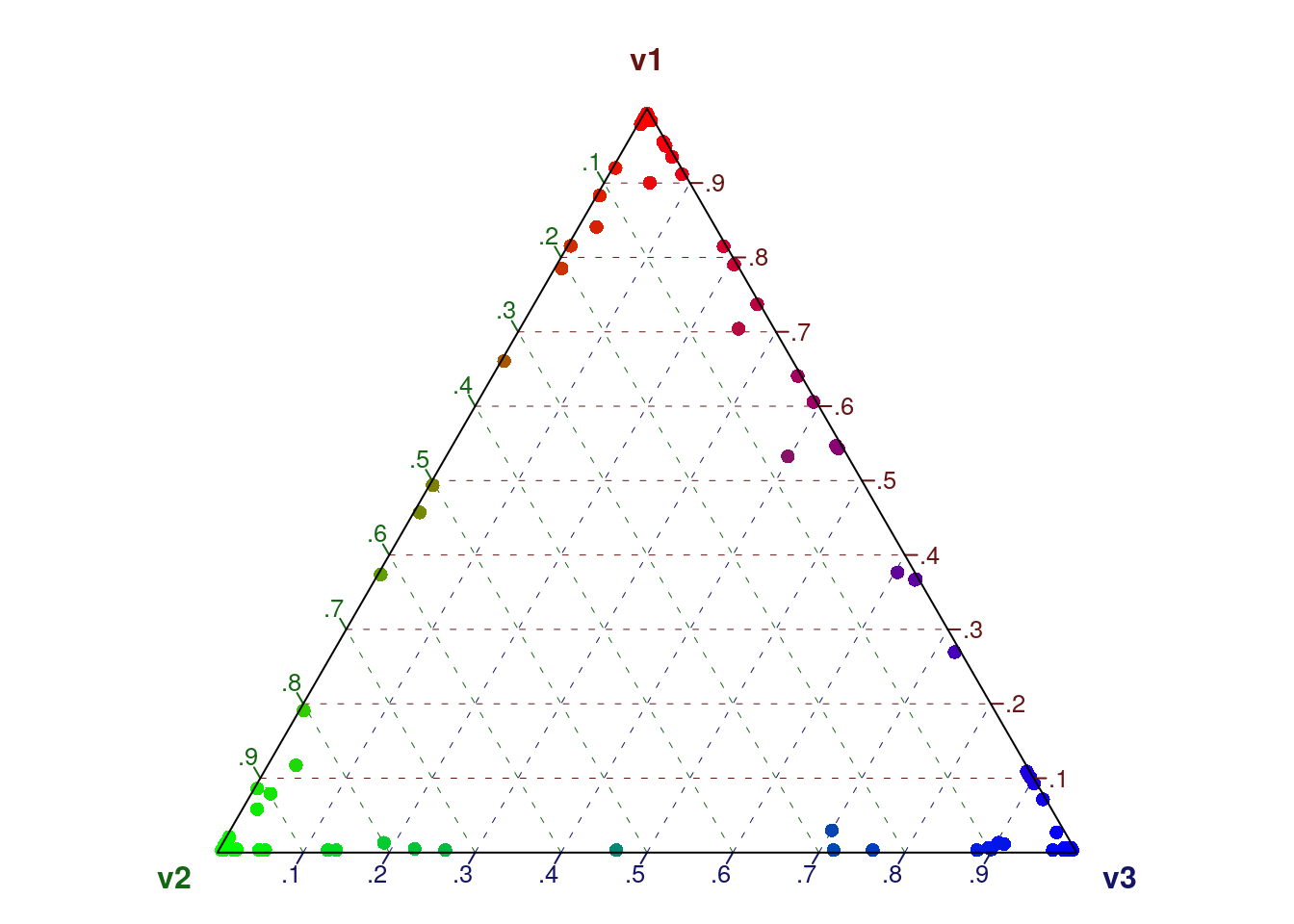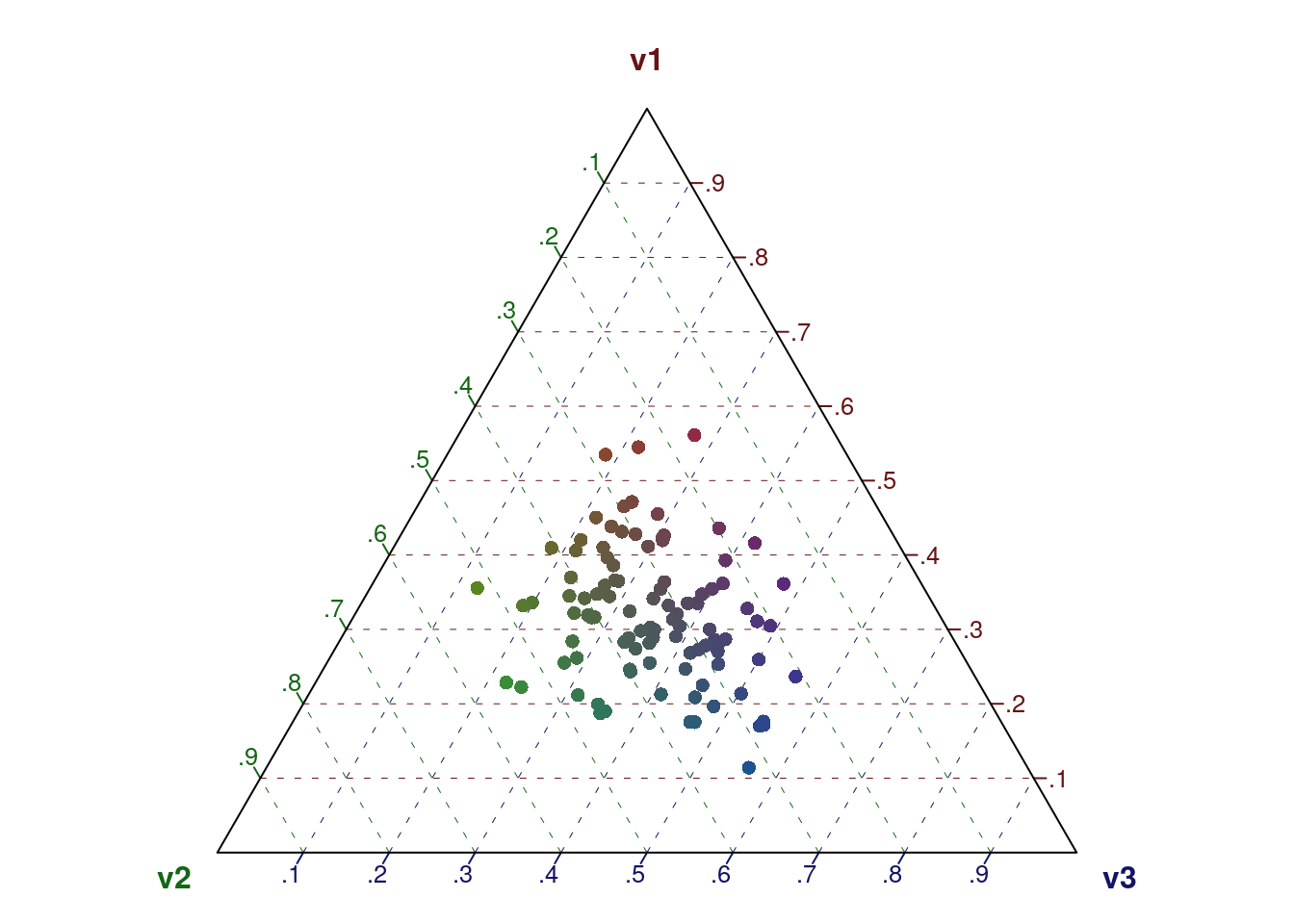# Dirichlet distributions, the alpha hyperparameter, and LDA

Kasper Welbers, VU University

### What is a dirichlet distribution?

A good way to think about a dirichlet distribution is as a distribution of multinomial distributions. For illustration, imagine a bag of dices. Each die has 6 sides, and the probability for each side to be trown can be described as a (single) multinomial distribution. The probability mass function (PMF) for each die is then a vector of length 6, that gives the probability for each side, and sums to 1. Now, given a bag of dices, you basically have a bag of PMF’s. The dirichlet distribution can be used to describe the distribution of these PMF’s. For example, if you were to take one die from the bag, how likely is it that you get a fair die, or a die that is more likely to trow a six?

### The alpha parameter

The dirichlet distribution has a single parameter, often referred to as the alpha parameter. This parameter determines both the distribution and concentration of the dirichlet.

If the alpha is a scalar (i.e. a single value), it only determines the concentration of the dirichlet. A higher alpha then gives a more dense distribution whereas a lower alpha gives a more sparse distribution. In the example of a bag of dices, a dense distribution means that each die in the bag is likely to have a faily uniform PMF. Thus, it’s a bag of fair dices. If the distribution is sparse, this means that many dices are skewed towards certain sides. Since the distribution is symmetrical, this skew towards certain sides is random.

If the alpha is a vector, then it determines both the concentration and distribution of the dirichlet. In the example of the bag of (six sided) dices, the alpha would be a vector of length 6, and a value in the vector would correspond to the number of eyes on one side of a die. If, for example, the values in the vector for the side with 6 eyes is higher, then the bag of dices is more likely to contain dices that are skewed towards trowing 6 eyes. The overal hight of the alpha still determines the concentration. How this works is best illustrated (we’ll get to the examples in a minute).

### This document

This document contains some examples of dirichlet distributions with different alpha parameters. It first shows this for the example of a bag of dices. Then, it shows how the dirichlet distribution can be visualized (which is a great way to play with the alpha parameter and see the consequences). Finally, it discusses the use of the dirichlet distribution and the alpha parameter in Latent Dirichlet Allocation.

# Example: the dirichlet distribution of a bag of dices

``install.packages('DirichletReg')``
``library(DirichletReg)``

To illustrate the effect of alpha on the dirichlet distribution, we generate some random dirichlet distributions with different alpha values.

In this example, we always give the alpha as a vector, because the rdirichlet function needs the length of the vector to determine K. However, if each value of the alpha vector is the same, it can actually be considered as a scalar. So, if alpha is the vector c(1,1,1), it is synoymous to using the scalar c(1) for a dirichlet distribution with K = 3.

### A bag of fair dices (dense and symmetric)

The rdirichlet function generates random numbers according to the dirichlet distribution. This is a matrix with K columns and N rows. Each row can be considered a die, with the columns representing the PMF of the die. In a bag of fair dices, we would thus expect the column means (i.e. the means across all dices) to be fairly uniform. Also, the standard deviation should be low, because we expect each die to be fair.

``````N = 1000
alpha = c(100,100,100,100,100,100)
diri = rdirichlet(N, alpha)
apply(diri, 2, 'mean')``````
``##  0.1666857 0.1664649 0.1662356 0.1667906 0.1675893 0.1662338``
``apply(diri, 2, 'sd')``
``##  0.01579785 0.01508251 0.01475499 0.01495452 0.01580631 0.01528022``

### A bag of bad dices, but the bag is fair in the sense there is no clear skew (sparse and symmetric)

If we lower the alpha, we get a more sparse concentration. Since the distribution is symmetric (we use the same value for all alpha, like a scalar) the means are still rather uniform. However, since each individual die is skewed, the standard deviations should be higher.

``````N = 1000
alpha = c(0.1,0.1,0.1,0.1,0.1,0.1)
diri = rdirichlet(N, alpha)
apply(diri, 2, 'mean')``````
``##  0.1470116 0.1729860 0.1631450 0.1826368 0.1745679 0.1596528``
``apply(diri, 2, 'sd')``
``##  0.2705492 0.2988804 0.2927545 0.3043417 0.3050061 0.2905302``

### A bag of false dices. If you draw a random dice, you are more likely to draw a dice that is rigged to throw a 6

Now we give a vector as alpha in which the sixth value is much higher. Thus, the mean for the sixth column is much higher. Also note that the standard deviation is low, because each die is heavily skewed towards 6.

``````N = 1000
alpha = c(0.1,0.1,0.1,0.1,0.1,100)
diri = rdirichlet(N, alpha)
apply(diri, 2, 'mean')``````
``````##  0.0011163090 0.0010794293 0.0010923234 0.0009881648 0.0009227075
##  0.9948010659``````
``apply(diri, 2, 'sd')``
``##  0.003626562 0.003226867 0.003118322 0.003096790 0.002785668 0.007059163``

### Two strange bags of false dices, to illustrate how only changing the concentration affects the dirichlet.

Bag 1 contains dices that are likely to trow either a 5 or a 6.

``````N = 1000
alpha1 = c(0.1,0.1,0.1,0.1,100,100)
diri1 = rdirichlet(N, alpha1)
``````##      [,1] [,2] [,3] [,4] [,5] [,6]
## [1,] 0.01 0.00    0    0 0.50 0.49
## [2,] 0.00 0.00    0    0 0.57 0.43
## [3,] 0.00 0.00    0    0 0.51 0.48
## [4,] 0.00 0.00    0    0 0.45 0.54
## [5,] 0.00 0.01    0    0 0.54 0.46
## [6,] 0.00 0.00    0    0 0.54 0.45``````

If we make the concentration more sparse by lowering the alpha, we get a very different outcome (note that we only divide the previous alpha by 1000, keeping the same distribution with only a different concentration). Bag 2 contains dices that are either skewed towards 5 OR skewed towards 6.

``````N = 1000
alpha2 = alpha1 / 1000 ## the previous alpha, but divided by 1000 to make distribution more dense
diri2 = rdirichlet(N, alpha2)
``````##      [,1] [,2] [,3] [,4] [,5] [,6]
## [1,]    0    0    0    0 0.87 0.13
## [2,]    0    0    0    0 0.39 0.61
## [3,]    0    0    0    0 1.00 0.00
## [4,]    0    0    0    0 0.00 1.00
## [5,]    0    0    0    0 0.00 1.00
## [6,]    0    0    0    0 0.94 0.06``````

# Visualization

We can visualize a dirichlet distribution nicely if we have a distribution with K=3 (or, an alpha of length 3). Since a PMF sums to 1, we can plot this in two dimenstions.

Low, symmetrical alpha: sparse and even distribution (each document often contains only 1 topic)

``````diri = rdirichlet(100, alpha = c(0.1,0.1,0.1))
dr = DR_data(diri) ## for visualization, make this a DirichletRegData object``````
``## Warning in DR_data(diri): some entries are 0 or 1 => transformation forced``
``plot(dr) ``high, symmetrical alpha: sense and even distribution (each document often contains a mixture of all topics)

``````diri = rdirichlet(100, alpha = c(10,10,10))
dr = DR_data(diri)
plot(dr) ``````Low, assymmetrical alpha: sparse and uneven distribution (2 high value topics more likely to occur, but often one OR the other. Due to sparseness, the low value topic can still be dominant in a few topics)

``````diri = rdirichlet(100, alpha = c(0.1,0.5,0.5))
dr = DR_data(diri) ``````
``## Warning in DR_data(diri): some entries are 0 or 1 => transformation forced``
``plot(dr) ``